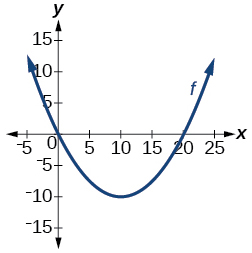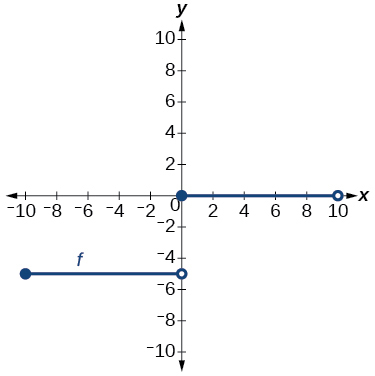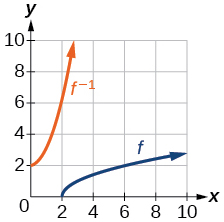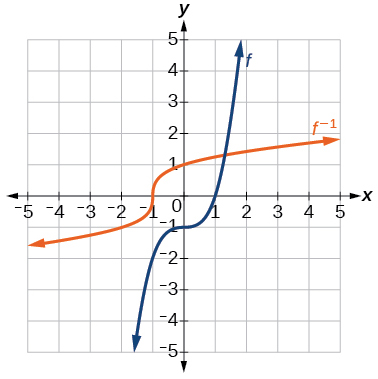3.7 Inverse functions  (Page 7/9)

 Page 7 / 9

For the following exercises, use function composition to verify that $\text{\hspace{0.17em}}f\left(x\right)\text{\hspace{0.17em}}$ and $\text{\hspace{0.17em}}g\left(x\right)\text{\hspace{0.17em}}$ are inverse functions.

$f\left(x\right)=\sqrt{x-1}\text{\hspace{0.17em}}$ and $\text{\hspace{0.17em}}g\left(x\right)={x}^{3}+1$

$f\left(x\right)=-3x+5\text{\hspace{0.17em}}$ and $\text{\hspace{0.17em}}g\left(x\right)=\frac{x-5}{-3}$

Graphical

For the following exercises, use a graphing utility to determine whether each function is one-to-one.

$f\left(x\right)=\sqrt{x}$

one-to-one

$f\left(x\right)=\sqrt{3x+1}$

$f\left(x\right)=-5x+1$

one-to-one

$f\left(x\right)={x}^{3}-27$

For the following exercises, determine whether the graph represents a one-to-one function.not one-to-oneFor the following exercises, use the graph of $\text{\hspace{0.17em}}f\text{\hspace{0.17em}}$ shown in [link] .

Find $\text{\hspace{0.17em}}f\left(0\right).$

$3$

Solve $\text{\hspace{0.17em}}f\left(x\right)=0.$

Find $\text{\hspace{0.17em}}{f}^{-1}\left(0\right).$

$2$

Solve $\text{\hspace{0.17em}}{f}^{-1}\left(x\right)=0.$

For the following exercises, use the graph of the one-to-one function shown in [link] .

Sketch the graph of $\text{\hspace{0.17em}}{f}^{-1}.\text{\hspace{0.17em}}$Find

If the complete graph of $\text{\hspace{0.17em}}f\text{\hspace{0.17em}}$ is shown, find the domain of $\text{\hspace{0.17em}}f.\text{\hspace{0.17em}}$

$\left[2,10\right]$

If the complete graph of $\text{\hspace{0.17em}}f\text{\hspace{0.17em}}$ is shown, find the range of $\text{\hspace{0.17em}}f.$

Numeric

For the following exercises, evaluate or solve, assuming that the function $\text{\hspace{0.17em}}f\text{\hspace{0.17em}}$ is one-to-one.

If $\text{\hspace{0.17em}}f\left(6\right)=7,\text{\hspace{0.17em}}$ find $\text{\hspace{0.17em}}\text{\hspace{0.17em}}{f}^{-1}\left(7\right).$

$6$

If $\text{\hspace{0.17em}}f\left(3\right)=2,\text{\hspace{0.17em}}$ find $\text{\hspace{0.17em}}{f}^{-1}\left(2\right).$

If $\text{\hspace{0.17em}}{f}^{-1}\left(-4\right)=-8,\text{\hspace{0.17em}}$ find $\text{\hspace{0.17em}}f\left(-8\right).$

$-4$

If $\text{\hspace{0.17em}}{f}^{-1}\left(-2\right)=-1,\text{\hspace{0.17em}}$ find $\text{\hspace{0.17em}}f\left(-1\right).$

For the following exercises, use the values listed in [link] to evaluate or solve.

$x$ $f\left(x\right)$
0 8
1 0
2 7
3 4
4 2
5 6
6 5
7 3
8 9
9 1

Find $\text{\hspace{0.17em}}f\left(1\right).$

$0$

Solve $\text{\hspace{0.17em}}f\left(x\right)=3.$

Find $\text{\hspace{0.17em}}{f}^{-1}\left(0\right).$

$\text{\hspace{0.17em}}1\text{\hspace{0.17em}}$

Solve $\text{\hspace{0.17em}}{f}^{-1}\left(x\right)=7.$

Use the tabular representation of $\text{\hspace{0.17em}}f\text{\hspace{0.17em}}$ in [link] to create a table for $\text{\hspace{0.17em}}{f}^{-1}\left(x\right).$

 $x$ 3 6 9 13 14 $f\left(x\right)$ 1 4 7 12 16
 $x$ 1 4 7 12 16 ${f}^{-1}\left(x\right)$ 3 6 9 13 14

Technology

For the following exercises, find the inverse function. Then, graph the function and its inverse.

$f\left(x\right)=\frac{3}{x-2}$

$f\left(x\right)={x}^{3}-1$

${f}^{-1}\left(x\right)={\left(1+x\right)}^{1/3}$Find the inverse function of $\text{\hspace{0.17em}}f\left(x\right)=\frac{1}{x-1}.\text{\hspace{0.17em}}$ Use a graphing utility to find its domain and range. Write the domain and range in interval notation.

Real-world applications

To convert from $\text{\hspace{0.17em}}x\text{\hspace{0.17em}}$ degrees Celsius to $\text{\hspace{0.17em}}y\text{\hspace{0.17em}}$ degrees Fahrenheit, we use the formula $\text{\hspace{0.17em}}f\left(x\right)=\frac{9}{5}x+32.\text{\hspace{0.17em}}$ Find the inverse function, if it exists, and explain its meaning.

${f}^{-1}\left(x\right)=\frac{5}{9}\left(x-32\right).\text{\hspace{0.17em}}$ Given the Fahrenheit temperature, $\text{\hspace{0.17em}}x,\text{\hspace{0.17em}}$ this formula allows you to calculate the Celsius temperature.

The circumference $\text{\hspace{0.17em}}C\text{\hspace{0.17em}}$ of a circle is a function of its radius given by $\text{\hspace{0.17em}}C\left(r\right)=2\pi r.\text{\hspace{0.17em}}$ Express the radius of a circle as a function of its circumference. Call this function $\text{\hspace{0.17em}}r\left(C\right).\text{\hspace{0.17em}}$ Find $\text{\hspace{0.17em}}r\left(36\pi \right)\text{\hspace{0.17em}}$ and interpret its meaning.

A car travels at a constant speed of 50 miles per hour. The distance the car travels in miles is a function of time, $\text{\hspace{0.17em}}t,\text{\hspace{0.17em}}$ in hours given by $\text{\hspace{0.17em}}d\left(t\right)=50t.\text{\hspace{0.17em}}$ Find the inverse function by expressing the time of travel in terms of the distance traveled. Call this function $\text{\hspace{0.17em}}t\left(d\right).\text{\hspace{0.17em}}$ Find $\text{\hspace{0.17em}}t\left(180\right)\text{\hspace{0.17em}}$ and interpret its meaning.

$t\left(d\right)=\frac{d}{50},\text{\hspace{0.17em}}$ $t\left(180\right)=\frac{180}{50}.\text{\hspace{0.17em}}$ The time for the car to travel 180 miles is 3.6 hours.

Functions and Function Notation

For the following exercises, determine whether the relation is a function.

$\left\{\left(a,b\right),\left(c,d\right),\left(e,d\right)\right\}$

function

$\left\{\left(5,2\right),\left(6,1\right),\left(6,2\right),\left(4,8\right)\right\}$

${y}^{2}+4=x,\text{\hspace{0.17em}}$ for $\text{\hspace{0.17em}}x\text{\hspace{0.17em}}$ the independent variable and $\text{\hspace{0.17em}}y\text{\hspace{0.17em}}$ the dependent variable

not a function

Is the graph in [link] a function?

For the following exercises, evaluate the function at the indicated values: $\text{\hspace{0.17em}}\text{\hspace{0.17em}}\text{\hspace{0.17em}}f\left(-3\right);\text{\hspace{0.17em}}\text{\hspace{0.17em}}f\left(2\right);\text{\hspace{0.17em}}\text{\hspace{0.17em}}\text{\hspace{0.17em}}f\left(-a\right);\text{\hspace{0.17em}}\text{\hspace{0.17em}}\text{\hspace{0.17em}}-f\left(a\right);\text{\hspace{0.17em}}\text{\hspace{0.17em}}\text{\hspace{0.17em}}f\left(a+h\right).$

$f\left(x\right)=-2{x}^{2}+3x$

$f\left(-3\right)=-27;$ $f\left(2\right)=-2;$ $f\left(-a\right)=-2{a}^{2}-3a;$
$-f\left(a\right)=2{a}^{2}-3a;$ $f\left(a+h\right)=-2{a}^{2}+3a-4ah+3h-2{h}^{2}$

$f\left(x\right)=2|3x-1|$

For the following exercises, determine whether the functions are one-to-one.

sin^4+sin^2=1, prove that tan^2-tan^4+1=0
what is the formula used for this question? "Jamal wants to save \$54,000 for a down payment on a home. How much will he need to invest in an account with 8.2% APR, compounding daily, in order to reach his goal in 5 years?"
i don't need help solving it I just need a memory jogger please.
Kuz
A = P(1 + r/n) ^rt
Dale
how to solve an expression when equal to zero
its a very simple
Kavita
gave your expression then i solve
Kavita
Hy guys, I have a problem when it comes on solving equations and expressions, can you help me 😭😭
Thuli
Tomorrow its an revision on factorising and Simplifying...
Thuli
ok sent the quiz
kurash
send
Kavita
Hi
Masum
What is the value of log-1
Masum
the value of log1=0
Kavita
Log(-1)
Masum
What is the value of i^i
Masum
log -1 is 1.36
kurash
No
Masum
no I m right
Kavita
No sister.
Masum
no I m right
Kavita
tan20°×tan30°×tan45°×tan50°×tan60°×tan70°
jaldi batao
Joju
Find the value of x between 0degree and 360 degree which satisfy the equation 3sinx =tanx
what is sine?
what is the standard form of 1
1×10^0
Akugry
Evalute exponential functions
30
Shani
The sides of a triangle are three consecutive natural number numbers and it's largest angle is twice the smallest one. determine the sides of a triangle
Will be with you shortly
Inkoom
3, 4, 5 principle from geo? sounds like a 90 and 2 45's to me that my answer
Neese
answer is 2, 3, 4
Gaurav
prove that [a+b, b+c, c+a]= 2[a b c]
can't prove
Akugry
i can prove [a+b+b+c+c+a]=2[a+b+c]
this is simple
Akugry
hi
Stormzy
x exposant 4 + 4 x exposant 3 + 8 exposant 2 + 4 x + 1 = 0
x exposent4+4x exposent3+8x exposent2+4x+1=0
HERVE
How can I solve for a domain and a codomains in a given function?
ranges
EDWIN
Thank you I mean range sir.
Oliver
proof for set theory
don't you know?
Inkoom
find to nearest one decimal place of centimeter the length of an arc of circle of radius length 12.5cm and subtending of centeral angle 1.6rad
factoring polynomialByByBy